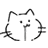# 抱歉，您的浏览器无法访问本站

## 记录7_QMZFWQ_2JMS4Z46F_T9.png

## 题解

### C2 - k-LCM (hard version)XJQ1VOCX5_3_D_4ML2KN.png

### D - Genius

$$1 \dots n$$$$n \leq 5000$$ 个位置：$$i$$-th 位置有 $$tag_i$$$$c_i = 2^i$$$$s_i$$ 分数；初始有 $$\mathrm{IQ} = 0$$$$S = 0$$

• $$tag_i \ne tag_j$$：这个简单，如果不满足直接阻止对应的转移就行了。
• $$\mathrm{IQ} < |c_i - c_j|$$：意思就是说走的边越来越长；因此我们总是先转移短边再转移长边就行。

### E2 - Square-free division (hard version)

• $$j > 0$$，那么显然可以从 $$F_{i,k} (k < j)$$ 转移到 $$F_{i, j}$$
• 若区间 $$(i, k]$$ 消耗了 $$x$$ 次修改而得以连续，那么 $$F_{k, j + x} = F_{i, j} + 1$$

## 后记

《就这》：比赛中：Codeforces 服务器就这？比赛后：你这通过情况就这？